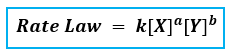# Problem: Consider this reaction and its rate law. 4A + 3B → products   rate = k[A] 2 [B]2.a) What is the order with respect to A?b) What is the order with respect to B?c) What is the overall reaction order?

###### FREE Expert Solution
88% (175 ratings)
###### FREE Expert Solution

Recall that the rate law only focuses on the reactant concentrations and has a general form of:k = rate constant

X & Y = reactants

a & b = reactant orders

88% (175 ratings)###### Problem Details

Consider this reaction and its rate law. 4A + 3B → products   rate = k[A] 2 [B]2.

a) What is the order with respect to A?

b) What is the order with respect to B?

c) What is the overall reaction order?

What scientific concept do you need to know in order to solve this problem?

Our tutors have indicated that to solve this problem you will need to apply the Rate Law concept. You can view video lessons to learn Rate Law. Or if you need more Rate Law practice, you can also practice Rate Law practice problems.

What is the difficulty of this problem?

Our tutors rated the difficulty ofConsider this reaction and its rate law. 4A + 3B → products ...as low difficulty.

How long does this problem take to solve?

Our expert Chemistry tutor, Dasha took 1 minute and 27 seconds to solve this problem. You can follow their steps in the video explanation above.

What professor is this problem relevant for?

Based on our data, we think this problem is relevant for Professor Polo's class at VCU.